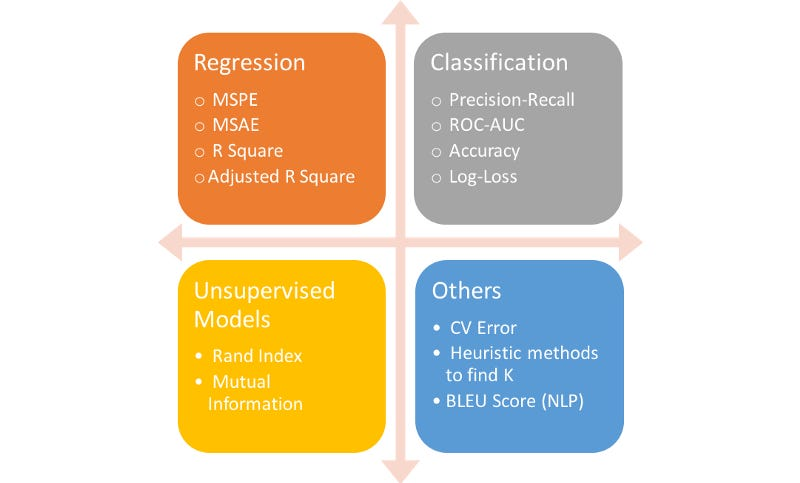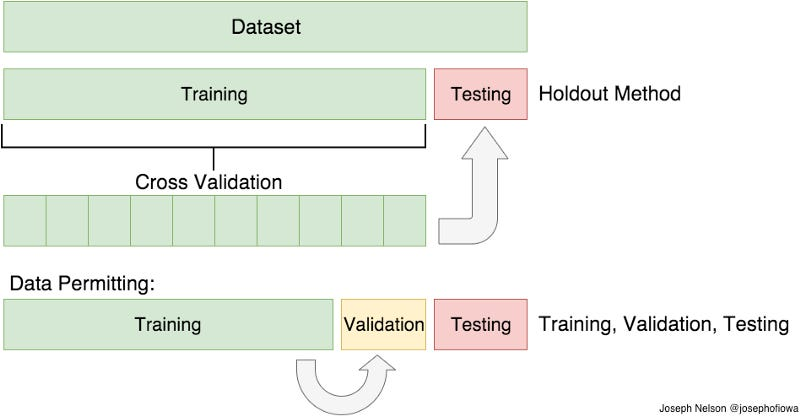# #06 Model Validation: Practical Metric Lists only you need to know

Source: Deep Learning on Medium

## モデルの評価指標まとめ

Hola! Welcome to #ShortcutML Series! Cheat Note for everyone!

### Target is who wanna know …

• Reason: Model Applying isn’t the end of ML
• Big Picture: Summarize too many validation metrics
• Code: The simplest python code for each preprocessing

— — —

### Why you have to read this?Choosing the Right Metric for Evaluating Machine Learning Models — Part 1, Alvira Swalin

Before we jump on the main topic, when do we evaluate our model? The answer is not only once. Generally, we use model validation metrics twice in our real Data Science workflow:

2. Model Improvement: with tuning hyperparameters

To get a more clear picture of the difference between these two, let me explain by the workflow of ML implementation. So after you set all the features X for your task y, you might prepare multiple ML models as candidates.

Then how can you finally choose one for your task? Yes, this is the first point when you use model validation metrics. Scikit-learn provides some shortcut methods to compare models like cross_validation.

Next, after you choose one particular model with the best accuracy, you will jump on Hyperparameter Tuning part to improve more accuracy and versatility. Here is the second point you’ll use these metrics.

In this article, I’m trying to make a Cheat Note of Model Evaluation Metrics. So let’s get started!

— — —

1. Cross-Validation
2. Metrics for Regression problem
3. Metrics for the Classification problem
4. Metrics for Clustering problem

### 1. Cross-Validation for model comparisonVisual Representation of Train/Test Split and Cross Validation. H/t to my DSI instructor, Joseph Nelson

The starting point of this why and how we split data is Generalization. Because our goal of building a machine learning model is real implementation with unknown data from the future. So we don’t need useless models which are overfitting with past data.

Therefore the biggest difference between these two methods is the way of handling “training data”. One is fix training data, but another is randomly and diversely picking up training data to create a more generalized model.

1. Holdout Method

`from sklearn.model_selection import train_test_splitfrom sklearn.datasets import load_winewine = load_wine()X, y = wine.data, wine.targetX_train, X_test, y_train, y_test = train_test_split(X, y, test_size=0.3)`

2. Cross-Validation Method

`# Decision Tree Classifieras for estimatorfrom sklearn.tree import DecisionTreeClassifierclf = DecisionTreeClassifier(random_state=0)`

2–1. cross_val_score: simplest coding method

We can decide the number of data splitting by a parameter “cv”. Normally 5 is considered as a standard splitting number.

`# X, y = wine.data, wine.targetfrom sklearn.model_selection import cross_val_scorescores = cross_val_score(clf, X, y, cv=5)print(scores) # cv = number of splited dataprint(scores.mean())`
`>>> array([0.78378378, 0.86111111, 0.88888889, 0.91428571, 0.85294118])>>> 0.86 # Accuracy was 86% (not enough!)`

2–1. cross_validate: I recommend this customizable one

`scoring = ['precision_macro', 'recall_macro']scores = cross_validate(clf, X, y, scoring=scoring, cv=5)print(scores)`
`>>> {'test_recall_macro': array([0.76666667, 0.85238095, 0.90079365, >>> 0.91137566, 0.88095238]), >>> 'test_precision_macro': array([0.79878618, 0.86602564, >>> 0.88888889, 0.91851852, 0.87777778]), >>> 'score_time': array([0.00279498, 0.00261092, 0.00165415, >>> 0.00270295, 0.0016489 ]), >>> 'fit_time': array([0.00161314, 0.00124598, 0.00124192, >>> 0.00087595, 0.00107622])}`

### 2. Metrics for Regression

TL;DR: In most case, we use R2 or RMSE.

I’ll use Boston House Price dataset.

`# Data Preparationfrom sklearn.datasets import load_bostonboston = load_boston()X, y = boston.data, boston.target`
`# Train data and Test data Splittingfrom sklearn.model_selection import train_test_splitX_train, X_test, y_train, y_test = train_test_split(X, y, test_size=0.3)`

Model 1: Linear Regression

`reg = LinearRegression()reg.fit(X_train, y_train)y_pred1 = reg1.predict(X_test)`

Model 2: Decision Tree Regressor

`from sklearn.tree import DecisionTreeRegressorreg2 = DecisionTreeRegressor(max_depth=3)reg2.fit(X_train, y_train)y_pred2 = reg2.predict(X_test)`

Now we are ready to evaluate our two models and chose one!

1. R2: Coefficient of Determination

`from sklearn.metrics import r2_scorer2_score(y_test, y_pred1) # Linear Regressionr2_score(y_test, y_pred2) # Decision Tree Regressor`
`>>> 0.693909.. >>> 0.693134.. # Decision Tree Regressor won!`

when to use:

2. MSE: Mean Square Error

`from sklearn.metrics import mean_squared_errormean_squared_error(y_test, y_pred)`
`>>> 23.873348..`

when to use:

3. RMSE: Root Mean Square Error

`import numpy as npnp.sqrt(mean_squared_error(y_test, y_pred))`
`>>> 4.886036..`

when to use:

4. MAE: Mean Absolute Error

`reg = LinearRegression()reg.fit(X_train, y_train)y_pred = reg.predict(X_test)mean_absolute_error(y_test, y_pred)`
`>>> 3.465279..`

when to use:

### 3. Metrics for Classification

Overall Picture for a classification problem

1. One vs. One Classification: e.g. Paid user or free
2. One vs. Rest Classification: e.g. Premium member or Paid or free

I’ll use Iris dataset as a multi-class classification problem.

`# Data Preparationfrom sklearn.datasets import load_irisiris = load_iris()X, y = iris.data, iris.target`
`# Train data and Test data Splittingfrom sklearn.model_selection import train_test_splitX_train, X_test, y_train, y_test = train_test_split(X, y, test_size=0.3)`

Model 1: SVM

`from sklearn.svm import SVCclf1 = SVC(kernel = 'linear', C = 0.01)clf1.fit(X_train, y_train)y_pred1 = clf1.predict(X_test)`

Model 2: Naive Bayes

`from sklearn.naive_bayes import GaussianNBclf2 = GaussianNB()clf2.fit(X_train, y_train)y_pred2 = clf2.predict(X_test)`

Now we are ready to evaluate our two models and chose one!

1. Accuracy:

`from sklearn.metrics import accuracy_scoreaccuracy_score(y_test, y_pred1)accuracy_score(y_test, y_pred2)`
`>>> 0.933333.. >>> 0.888888.. # Gaussian Naive Bayes won!`

when to use:

2. Precision:

`from sklearn.metrics import precision_scoreprecision_score(y_test, y_pred1, average=None)precision_score(y_test, y_pred2, average=None)`
`>>> array([1. , 0.875 , 0.92307692])>>> array([1. , 0.88235294, 1. ]) # again GNB won!`

when to use:

3. Recall or Sensitivity:

`from sklearn.metrics import recall_scorerecall_score(y_test, y_pred2, average=None)`
`>>> array([1. , 1. , 0.85714286]) # GNB`

when to use:

4. F Score:

`from sklearn.metrics import f1_scoref1_score(y_test, y_pred2, average=None)`
`>>> array([1. , 0.9375 , 0.92307692]) # GNB`

when to use:

5. Confusion Matrix

`from sklearn.metrics import confusion_matrixconfusion_matrix(y_test, y_pred2)`
`>>> array([[16, 0, 0], [ 0, 15, 0], [ 0, 2, 12]]) # GNB`

when to use:

6. ROC: Receiver Operating Characteristic Curve

If you don’t use OneVsRestClassifier, it doesn’t work…

`from sklearn.multiclass import OneVsRestClassifierfrom sklearn.svm import LinearSVCclf = OneVsRestClassifier(LinearSVC(random_state=0))clf.fit(X_train, y_train)y_pred = clf.predict(X_test)`

Now we will check by ROC Curve.

`from sklearn.metrics import roc_curvefpr, tpr, thresholds = roc_curve(y_test, y_pred, pos_label=2)fpr, tpr, thresholds`
`>>> array([0. , 0.1 , 0.53333333, 1. ]) # False-Positive Rate>>> array([0., 1., 1., 1.]) # True-Positive Rate>>> array([3, 2, 1, 0]) # thresholds`

when to use:

7. AUC: Area Under Curve

`from sklearn.metrics import aucauc(fpr, tpr)`
`>>> 0.913333... # auc`

when to use:

8. Multi-class logarithmic loss

It’s a probability. And need to use OneVsRestClassifier.

`# clf = OneVsRestClassifier(LinearSVC(random_state=0))from sklearn.metrics import log_lossy_pred = clf.predict_proba(X_test) # not .predict()log_loss(y_test, y_pred)`
`>>> 0.09970990582482485`

when to use:

### 4. Metrics for Clustering

Basically in real clustering task, (I mean unsupervised clustering), we don’t have any method to measure accuracy or precision because nobody knows.

However as a process of classification task, sometimes we use supervised clustering to know the character of data. (In a real job as well.)

So I’ll quickly introduce some metrics for supervised clustering, in order to let you know just their existence (priority is very low though).

OK, I used only features from Iris dataset for a clustering problem.

`from sklearn.datasets import load_irisiris = load_iris()X, y = iris.data, iris.target`

As a representative model for a clustering problem, This time I used K-means.

`from sklearn.cluster import KMeanskmeans = KMeans(n_clusters=3, random_state=0)kmeans.fit(X)y_means = kmeans.predict(X)`

Now the result of supervised clustering is In y_means.

`# visulaliza result!plt.scatter(X[y_means==0,0],X[y_means==0,1],s=50, c='orange',label='Cluster1')plt.scatter(X[y_means==1,0],X[y_means==1,1],s=50, c='navy',label='Cluster2')plt.scatter(X[y_means==2,0],X[y_means==2,1],s=50, c='green',label='Cluster3')plt.scatter(kmeans.cluster_centers_[:,0], kmeans.cluster_centers_[:,1],s=200,marker='s', c='red', alpha=0.6, label='Centroids')plt.title('Iris segments')plt.show()`

1. Homogeneity score, Completeness Score, V-measure Score

`from sklearn.metrics import homogeneity_score, completeness_score, v_measure_scorehg = homogeneity_score(y, y_means) co = completeness_score(y, y_means)vm = v_measure_score(y, y_means)print(hg, co, vm)`
`>>> 0.751485..>>> 0.764986..>>> 0.758175..`

### 5. Additional: Learning Curve Visualization

`from sklearn.model_selection import learning_curvefrom sklearn.model_selection import ShuffleSplit`
`def plot_learning_curve(clf, title, X, y, ylim=None, cv=None, n_jobs=None, train_sizes=np.linspace(.1, 1.0, 5)): plt.figure() plt.title(title) if ylim is not None: plt.ylim(*ylim) plt.xlabel("Training examples") plt.ylabel("Score") train_sizes, train_scores, test_scores = learning_curve( clf, X, y, cv=cv, n_jobs=n_jobs, train_sizes=train_sizes) train_scores_mean = np.mean(train_scores, axis=1) train_scores_std = np.std(train_scores, axis=1) test_scores_mean = np.mean(test_scores, axis=1) test_scores_std = np.std(test_scores, axis=1) plt.grid()`
`plt.fill_between(train_sizes, train_scores_mean - train_scores_std, train_scores_mean + train_scores_std, alpha=0.1, color="r") plt.fill_between(train_sizes, test_scores_mean - test_scores_std, test_scores_mean + test_scores_std, alpha=0.1, color="g") plt.plot(train_sizes, train_scores_mean, 'o-', color="r", label="Training score") plt.plot(train_sizes, test_scores_mean, 'o-', color="g", label="Cross-validation score")`
`plt.legend(loc="best") return plt`
`title = "Learning Curves (Decision Tree, max_depth=2)"cv = ShuffleSplit(n_splits=100, test_size=0.2, random_state=0)`
`clf = DecisionTreeClassifier(max_depth=2, random_state=0)plot_learning_curve(clf, title, X, y, ylim=(0.7, 1.01), cv=cv, n_jobs=4)`
`title = "Learning Curves (SVM, Decision Tree, max_depth=5)"cv = ShuffleSplit(n_splits=10, test_size=0.2, random_state=0)clf = DecisionTreeClassifier(max_depth=5, random_state=0)plot_learning_curve(clf, title, X, y, (0.7, 1.01), cv=cv, n_jobs=4)`
`plt.show()`